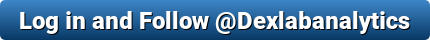# Using R Programming to Simulate the Incredible Pong Arcade Game

Unleashed in the market in 1972, Pong is one of the first computer games ever developed. Loosely inspired by tennis, Pong captured the worldwide gaming market soon after its launch. Instantaneously, it became a trending fad. Gaming enthusiasts became intrigued, they desired to delve deeper into the computer coding and system mechanisms mostly to understand the essence of arcade game development.

Today, R-Programming is extensively used to develop numerous board games. But the question to ponder on is – can we create traditional arcade games with R programming?

Well, of course we can! In this blog, we have recreated a 1980s arcade game but with R. The code written below simulates the historic game of Pong.The code applied here is based on Wacko’s Boing Program taken from the 1983 book Dr. C. Wacko’s Miracle Guide to Designing and Programming your own Atari Computer Arcade Games.  This book is marvellous! By clearly explaining how to write computer games using Atari Basic, the book is fun to read. It has a humorous tone, and the readers devour it.

The R code is well commented and seems to have a mind of its own! However, please note, the animation is a bit gawky, when it’s run in RStudio. For better animation, try to run it in native R Terminal.Now, let’s take a step closer to coding R-Cade game:

Video source – r-bloggers.com

### The Pong Code

```# Sound library
library(beepr)

# Game parameters
skill <- 0.87 # Skill (0-1)
score <- 0
high.score <- 0

# Define playing field
par(mar = rep(1,4), bg = "black")
plot.new()
plot.window(xlim = c(0, 30), ylim = c(0, 30))
lines(c(1, 30, 30, 1), c(0, 0, 30, 30), type = "l", lwd = 5, col = "white")

# Playing field boundaries (depend on cex)
xmin <- 0.5
xmax <- 29.4
ymin <- 0.5
ymax <- 29.4

# initial position
x <- sample(5:25, 1)
y <- sample(5:25, 1)
points(x, y, pch = 15, col = "white", cex = 2)

psize <- 4

# Set direction
dx <- runif(1, .5, 1)
dy <- runif(1, .5, 1)

# Game play
while (x > xmin - 1) {
sound <- 0 # Silence
Sys.sleep(.05) # Pause screen. Reduce to increase speed
points(x, y, pch = 15, col = "black", cex = 2) # Erase ball
# Move ball
x <- x + dx
y <- y + dy
# Collision detection
if (x > xmax) {
dx <- -dx * runif(1, .9, 1.1) # Bounce
if (x > xmin) x <- xmax # Boundary
sound <- 10 # Set sound
}
if (y < ymin | y > ymax) {
if (y < ymin) y <- ymin
if (y > ymax) y <- ymax
dy <- -dy * runif(1, .9, 1.1)
sound <- 10
}
if (x < xmin & (y > ypaddle - (psize / 2)) & y < ypaddle + (psize / 2)) {
if (x < xmin) x <- xmin
dx <- -dx * runif(1, .9, 1.1)
sound <- 2
score <- score + 1
}
# Draw ball
points(x, y, pch = 15, col = "white", cex = 2)
if (sound !=0) beep(sound)
if (runif(1, 0, 1) < skill) ypaddle <- ypaddle + dy # Imperfect follow
# Erase back line
lines(c(0, 0), c(0, 30), type = "l", lwd = 8, col = "black")
if (ypaddle > 30 - (psize / 2)) ypaddle <- 30 - (psize / 2)
lines(c(0, 0), c(ypaddle - (psize / 2), ypaddle + (psize / 2)), type = "l", lwd = 8, col = "white")
}
beep(8)
text(15,15, "GAME OVER", cex=5, col = "white")
s <- ifelse(score == 1, "", "s")
text(15,5, paste0(score, " Point", s), cex=3, col = "white")
```

Aspiring to become a data scientist? DexLab Analytics, a leading R programming training institute takes pride in offering a bouquet of R programming courses in Gurgaon. Log on to peruse through our intensive courses!

This post originally appeared onblogs.sas.com/content/iml/2017/05/15/intck-intnx-intervals-sas.html

#### Interested in a career in Data Analyst?### Call us to know more### Gurgaon+91 931 572 5902